Courses

# Performance of Transmission Lines, Line Parameters & Corona of Power Systems

## 10 Questions MCQ Test GATE Electrical Engineering (EE) 2022 Mock Test Series | Performance of Transmission Lines, Line Parameters & Corona of Power Systems

Description
This mock test of Performance of Transmission Lines, Line Parameters & Corona of Power Systems for Electrical Engineering (EE) helps you for every Electrical Engineering (EE) entrance exam. This contains 10 Multiple Choice Questions for Electrical Engineering (EE) Performance of Transmission Lines, Line Parameters & Corona of Power Systems (mcq) to study with solutions a complete question bank. The solved questions answers in this Performance of Transmission Lines, Line Parameters & Corona of Power Systems quiz give you a good mix of easy questions and tough questions. Electrical Engineering (EE) students definitely take this Performance of Transmission Lines, Line Parameters & Corona of Power Systems exercise for a better result in the exam. You can find other Performance of Transmission Lines, Line Parameters & Corona of Power Systems extra questions, long questions & short questions for Electrical Engineering (EE) on EduRev as well by searching above.
QUESTION: 1

### A sample of copper wire has a resistance of 50 Ω at 100C. The maximum operating temperature of the wire if its resistance is to increase by at most 10 percent will be: (Given, the Temperature coefficient at 100C is a = 0.004090C-1).

Solution: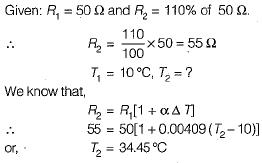QUESTION: 2

### A single-phase, two wire transmission line, 15 km long, is made up of round conductors, each 0.8 cm radius, separated from each other by 40 cm. The equivalent diameter of a fictitious hollow, thin-walled conductor having the same inductance as the original line is given by

Solution:

The fictitious conductor is one whose radius is r' and whose diameter is therefore,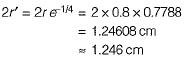QUESTION: 3

### If the conductor radius increases, inductance of the line,

Solution:

Inductance,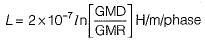Here, GMR radius of conductor.
Hence, if radius increases, GMR also increases. As a result of which inductance will decrease.

QUESTION: 4

For an underground cable, the surge impedance is generally taken as

Solution:

For underground cable, Zs = 40 Ω
For overhead line, Zs = 400 Ω

QUESTION: 5

Consider the following phenomenon occuring in a power system:
1. Corona loss
2. Charging current
3. Ferranti effect
4. Dielectric loss
5. Skin effect
Which of the above are associated with transmission lines?

Solution:

Dielectric loss occurs in underground cables not in a transmission line.

QUESTION: 6

The corona loss or the critical disruptive voltage in a transmission line at a temperature (f) and pressure (b) can be foundout by Using “air density correction factor (δ)” given by

Solution:
QUESTION: 7

A three-phase, 50 Hz, 400 kV, transmission line is 300 km long. The line inductance is 0.97 mH/km per phase and capacitance is 0.0115 μF/km per phase. Assuming a lossless line, the surge impedance of the line will be

Solution:

Surge impedance of the line is given by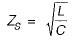or,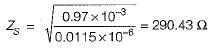QUESTION: 8

The resistance and reactance of a short line are equal. At zero regulation the load will be

Solution:

Zero regulation of a transmission line occurs at a leading power factor when φ = θ.
Since R = X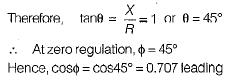QUESTION: 9

Shunt reactors are needed

Solution:

Shunt reactors are used to bring down receiving end voltage during light load or no-load condition to overcome Ferranti effect.

QUESTION: 10

Operating transmission lines close to their maximum power transfer capability will impair

Solution: